Related Articles

# Seaborn – Sort Bars in Barplot

• Last Updated : 12 Nov, 2020

Prerequisite: Seaborn, Barplot

In this article, we are going to see how to sort the bar in barplot using Seaborn in python.

Seaborn is an amazing visualization library for statistical graphics plotting in Python. It provides beautiful default styles and color palettes to make statistical plots more attractive. It is built on the top of matplotlib library and also closely integrated to the data structures from pandas.

### Approach:

• Import module.
• Create a Dataframe.
• Create a barplot.
• Sort the Dataframe column with DataFrame.sort_values().
• Display the sort Dataframe into the barplot.

### So, Let’s implement to sort bar in barplot using seaborn with steps based on the above approach.

Step 1: Import required packages.

## Python3

 `# Import module``import` `pandas as pd``import` `numpy as np``import` `matplotlib.pyplot as plt``import` `seaborn as sns`

Step 2: Create a Dataframe to create a barplot.

## Python3

 `# Initialize data``State ``=` `[``"Rajasthan"``, ``"Bihar"``, ``"Madhya Pradesh"``,``         ``"Gujarat"``, ``"Maharashtra"``]``growth ``=` `[``342239``, ``94163``, ``308245``, ``196024``, ``307713``]`` ` `# Create a pandas dataframe``df ``=` `pd.DataFrame({``"State"``: State,``                   ``"Growth"``: growth})`` ` `# Display Dataframe``df`

Output: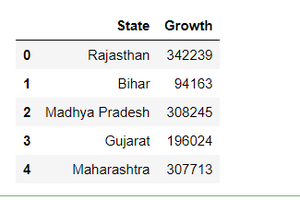Step 3: Create a barplot with this Dataframe.

## Python3

 `# make barplot``sns.barplot(x``=``'State'``, y``=``"Growth"``, data``=``df)`

Output: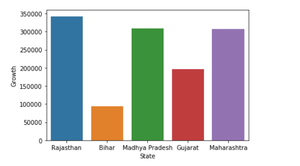Step 4: Let’s sort the Dateframe column(Growth column) with DataFrame.sort_values().

## Python3

 `# sort dataframe``df.sort_values(``'Growth'``)`

Output: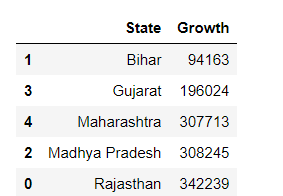We can do this same technique in a barplot.

## Python3

 `# make barplot and sort bars``sns.barplot(x``=``'State'``,``            ``y``=``"Growth"``, data``=``df, ``            ``order``=``df.sort_values(``'Growth'``).State)`

Output: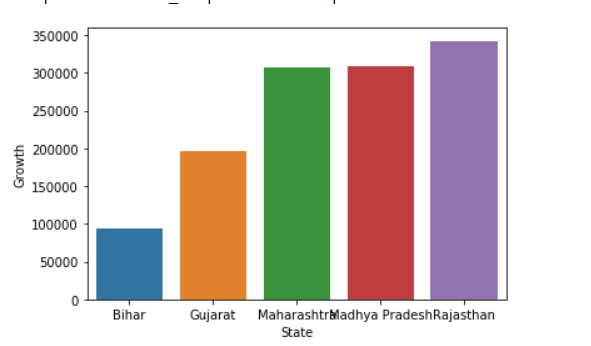Step 5: Let sort the bar with Descending order.

## Python3

 `# make barplot and sort bars``sns.barplot(x``=``'State'``,``            ``y``=``"Growth"``, data``=``df, ``            ``order``=``df.sort_values(``'Growth'``,ascending ``=` `False``).State)`

Output: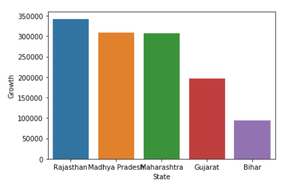Note: Default value of ascending is always True, if we change this parameter with False then it means that its arrange in descending order.

Below are the complete examples:

Example 1

## Python3

 `# import module``import` `pandas as pd``import` `numpy as np``import` `matplotlib.pyplot as plt``import` `seaborn as sns`` ` ` ` `# Initialize data``State ``=` `[``"Rajasthan"``, ``"Bihar"``, ``"Madhya Pradesh"``,``         ``"Gujarat"``, ``"Maharashtra"``]``growth ``=` `[``342239``, ``94163``, ``308245``, ``196024``, ``307713``]`` ` ` ` `# Create a pandas dataframe``df ``=` `pd.DataFrame({``"State"``: State,``                   ``"Growth"``: growth})`` ` ` ` `# sort dataframe``df.sort_values(``'Growth'``)`` ` ` ` `# make barplot and sort bars``sns.barplot(x``=``'State'``,``            ``y``=``"Growth"``, data``=``df, ``            ``order``=``df.sort_values(``'Growth'``).State)`

Output:Example 2

## Python3

 `# import module``import` `pandas as pd``import` `numpy as np``import` `matplotlib.pyplot as plt``import` `seaborn as sns`` ` ` ` `# Initialize data``State ``=` `[``"Rajasthan"``, ``"Bihar"``, ``"Madhya Pradesh"``,``         ``"Gujarat"``, ``"Maharashtra"``]``growth ``=` `[``342239``, ``94163``, ``308245``, ``196024``, ``307713``]`` ` ` ` `# Create a pandas dataframe``df ``=` `pd.DataFrame({``"State"``: State,``                   ``"Growth"``: growth})`` ` ` ` `# sort dataframe``df.sort_values(``'Growth'``)`` ` ` ` `# make barplot and sort bars``sns.barplot(x``=``'State'``,``            ``y``=``"Growth"``, data``=``df, ``            ``order``=``df.sort_values(``'Growth'``,ascending ``=` `False``).State)`

Output:Attention geek! Strengthen your foundations with the Python Programming Foundation Course and learn the basics.

To begin with, your interview preparations Enhance your Data Structures concepts with the Python DS Course. And to begin with your Machine Learning Journey, join the Machine Learning – Basic Level Course

My Personal Notes arrow_drop_up